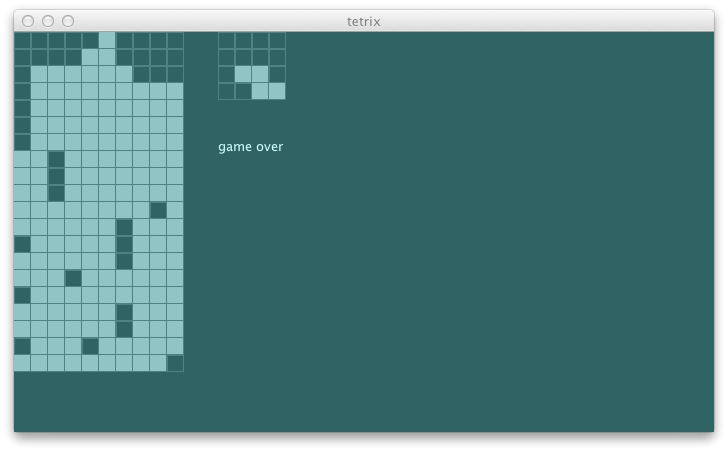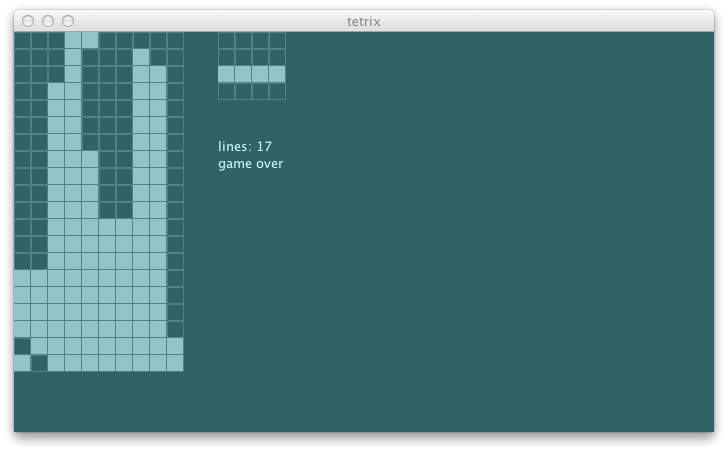# tetrix in Scala: day 9

Yesterday we got the tetrix-solving agent to play a better game by fixing the heuristic function and evaluating 36 possible positions of the current piece.

### stop watch

Let's start by creating a function to measure the time it takes to run a segment of code:

```  private[this] def stopWatch[A](name: String)(arg: => A): A = {
val t0 = System.currentTimeMillis
val retval: A = arg
val t1 = System.currentTimeMillis
println(name + " took " + (t1 - t0).toString + " ms")
retval
}```

This can be called as follows:

```    stopWatch("bestMove") {
// do something
} // stopWatch```

This outputs as follows:

```[info] Running com.tetrix.swing.Main
[info] bestMove took 84 ms
[info] selected List(MoveLeft, MoveLeft, MoveLeft, MoveLeft) -0.3
[info] bestMove took 43 ms
[info] selected List(MoveLeft, MoveLeft, MoveLeft) -0.3
[info] bestMove took 24 ms
[info] selected List(MoveLeft, MoveLeft) -0.3
[info] bestMove took 22 ms
[info] selected List(MoveLeft) -0.3
[info] bestMove took 26 ms
[info] selected List() -0.3
[info] bestMove took 17 ms
[info] selected List() -0.3
[info] bestMove took 10 ms
[info] selected List() -0.3
[info] bestMove took 8 ms
[info] selected List() -0.3
[info] bestMove took 11 ms
[info] selected List() -0.3
[info] bestMove took 13 ms
[info] selected List() -0.3```

There's the initial wind down as JIT compiler kicks in, and it eventually settles around 10 ms. This gives us roughly what kind of time frame we are dealing with.

### second level

If we expanded the search tree to the second piece, it should roughly increase 36x fold. 360 ms is not bad. Let's try this:

```  def bestMove(s0: GameState): StageMessage = {
var retval: Seq[StageMessage] = Nil
var current: Double = minUtility
stopWatch("bestMove") {
val nodes = actionSeqs(s0) map { seq =>
val ms = seq ++ Seq(Drop)
val s1 = Function.chain(ms map {toTrans})(s0)
val u = utility(s1)
if (u > current) {
current = u
retval = seq
} // if
SearchNode(s1, ms, u)
}
nodes foreach { node =>
actionSeqs(node.state) foreach { seq =>
val ms = seq ++ Seq(Drop)
val s2 = Function.chain(ms map {toTrans})(node.state)
val u = utility(s2)
if (u > current) {
current = u
retval = node.actions ++ seq
} // if
}
}
} // stopWatch
println("selected " + retval + " " + current.toString)
}```

Now it is starting to play much better. The thinking time ranged from 12 ms to 727 ms, but mostly they were around 100 or 200 ms.I am now comfortable enough to letting it drop the pieces again:

```class AgentActor(stageActor: ActorRef) extends Actor {
private[this] val agent = new Agent

def receive = {
case BestMove(s: GameState) =>
val message = agent.bestMove(s)
stageActor ! message
}
}```

### line number

Let's display the number of deleted lines on the swing UI.

```    val unit = blockSize + blockMargin
g drawString ("lines: " + view.lineCount.toString, 12 * unit, 7 * unit)```

Having this should help us track if our changes are improving the performance of the agent or not.### cavity

One thing I've been noticing as the agent plays the game is that it is happy to make cavities. A cavity is created when one or more block exists above an unfilled spot.

```-fig 1----

xxxx
xxxx x xxx
x x xxxxxx
----------```

Likely to avoid the height penalty, it would for example lay out the I bar flat on top of uneven surface instead of dropping it upright. To minimize the cavity, I'd like it to play:

```-fig 2-----

x
x  xxxx
x x xxx
x xxxxxxxx
----------```

Let's cauculate the height penalties from the first four columns.

```scala> val fig1 = math.sqrt(List(2, 2, 2, 2) map { x => x * x } sum)
fig1: Double = 4.0

scala> val fig2 = math.sqrt(List(1, 0, 4, 1) map { x => x * x } sum)
fig2: Double = 4.242640687119285```

As predicted, fig1 will incur lower penalty. We can create additional penalty for all blocks covering another block using its height * height. Then the new penalty becomes as follows:

```scala> val fig1b = math.sqrt(List(2, 2, 2, 2, 2, 2) map { x => x * x } sum)
fig1b: Double = 4.898979485566356

scala> val fig2b = math.sqrt(List(1, 0, 4, 1) map { x => x * x } sum)
fig2b: Double = 4.242640687119285```

This time, fig2 is preferred. Let's write this in spec:

```  def penalty2 = {
val s = newState(Seq(
(0, 0), (2, 0), (0, 1), (1, 1), (2, 1), (3, 1))
map { Block(_, TKind) }, (10, 20), TKind :: TKind :: Nil)
agent.penalty(s) must beCloseTo(4.89, 0.01)
}```

The test fails as expected:

```[info] Penalty function should
[info] + penalize having blocks stacked up high
[error] x penalize having blocks covering other blocks
[error]    4.0 is not close to 4.89 +/- 0.01 (AgentSpec.scala:13)```

Let's implement this using the REPL:

```scala>     val s = newState(Seq(
(0, 0), (2, 0), (0, 1), (1, 1), (2, 1), (3, 1))
map { Block(_, TKind) }, (10, 20), TKind :: TKind :: Nil)
s: com.eed3si9n.tetrix.GameState = GameState(List(Block((0,0),TKind), Block((2,0),TKind), Block((0,1),TKind), Block((1,1),TKind), Block((2,1),TKind), Block((3,1),TKind), Block((4,18),TKind), Block((5,18),TKind), Block((6,18),TKind), Block((5,19),TKind)),(10,20),Piece((5.0,18.0),TKind,List((-1.0,0.0), (0.0,0.0), (1.0,0.0), (0.0,1.0))),Piece((2.0,1.0),TKind,List((-1.0,0.0), (0.0,0.0), (1.0,0.0), (0.0,1.0))),List(),ActiveStatus,0)

scala> val groupedByX = s.unload(s.currentPiece).blocks map {_.pos} groupBy {_._1}
groupedByX: scala.collection.immutable.Map[Int,Seq[(Int, Int)]] = Map(3 -> List((3,1)), 1 -> List((1,1)), 2 -> List((2,0), (2,1)), 0 -> List((0,0), (0,1)))

scala> groupedByX map { case (k, vs) => vs.map(_._2).sorted.zipWithIndex }
res14: scala.collection.immutable.Iterable[Seq[(Int, Int)]] = List(List((1,0)), List((1,0)), List((0,0), (1,1)), List((0,0), (1,1)))

scala> groupedByX map { case (k, vs) => vs.map(_._2).sorted.zipWithIndex.dropWhile(x => x._1 == x._2) }
res15: scala.collection.immutable.Iterable[Seq[(Int, Int)]] = List(List((1,0)), List((1,0)), List(), List())

scala> val coverups = groupedByX flatMap { case (k, vs) => vs.map(_._2).sorted.zipWithIndex.dropWhile(x => x._1 == x._2).map(_._1 + 1) }
coverups: scala.collection.immutable.Iterable[Int] = List(2, 2)```

Here's the resulting `penalty`:

```  def penalty(s: GameState): Double = {
val groupedByX = s.unload(s.currentPiece).blocks map {_.pos} groupBy {_._1}
val heights = groupedByX map { case (k, v) => v.map({_._2 + 1}).max }
val coverups = groupedByX flatMap { case (k, vs) =>
vs.map(_._2).sorted.zipWithIndex.dropWhile(x => x._1 == x._2).map(_._1 + 1) }
math.sqrt( (heights ++ coverups) map { x => x * x } sum)
}```

This passes the test:

```[info] Penalty function should
[info] + penalize having blocks stacked up high
[info] + penalize having blocks covering other blocks```

Let's see how it plays:The cavity avoidance is working, but almost too well. Maybe we should reinstate the gap penalty tomorrow.

```\$ git fetch origin
\$ git co day9 -b try/day9
\$ sbt "project swing" run```